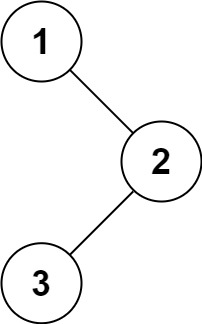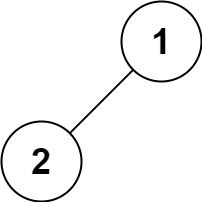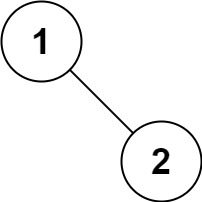GeetCode Hub

Given the root of a binary tree, return the inorder traversal of its nodes' values.

Example 1:Input: root = [1,null,2,3]
Output: [1,3,2]

Example 2:

Input: root = []
Output: []

Example 3:

Input: root = 
Output: 

Example 4:Input: root = [1,2]
Output: [2,1]

Example 5:Input: root = [1,null,2]
Output: [1,2]

Constraints:

• The number of nodes in the tree is in the range [0, 100].
• -100 <= Node.val <= 100

Follow up: Recursive solution is trivial, could you do it iteratively?

/** * Definition for a binary tree node. * public class TreeNode { * int val; * TreeNode left; * TreeNode right; * TreeNode() {} * TreeNode(int val) { this.val = val; } * TreeNode(int val, TreeNode left, TreeNode right) { * this.val = val; * this.left = left; * this.right = right; * } * } */ class Solution { public List<Integer> inorderTraversal(TreeNode root) { } }